Learning Library
Who we are
Guidance

# 1.OA.A.1 Worksheets, Workbooks, Lesson Plans, and Games

#### CCSS.maths.CONTENT.1.OA.A.1

:
"Use addition and subtraction within 20 to solve word problems involving situations of adding to, taking from, putting together, taking apart, and comparing, with unknowns in all positions, e.g., by using objects, drawings, and equations with a symbol for the unknown number to represent the problem."

Our Take:When solving word problems that involve addition and subtraction within 20, the first grader will use grade-appropriate strategies, such as writing an equation with a symbol for the unknown and drawing a picture.

These worksheets, games, and lesson plans can help students practise this Common Core State Standards skill.

## Worksheets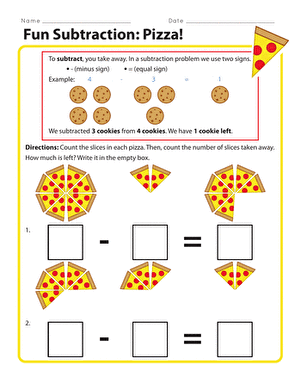Fun Subtraction: Pizza!
Worksheet
Fun Subtraction: Pizza!
In this fun subtraction worksheet, your child will need to count the number of pizza slices, how many slices were eaten, and make a simple subtraction problem.
Year 2
Maths
Worksheet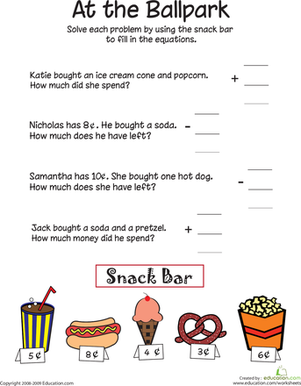At the Ballpark: Addition and Subtraction
Worksheet
At the Ballpark: Addition and Subtraction
Even at the ballpark, solving story problems is important. How much will it cost to buy a hot dog and an ice cream cone?
Year 2
Maths
Worksheet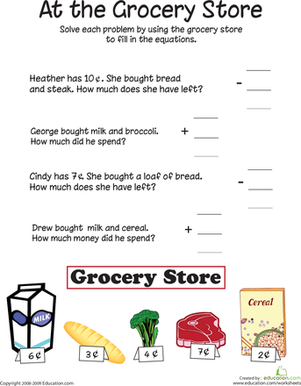At the Grocery Store: Addition and Subtraction
Worksheet
At the Grocery Store: Addition and Subtraction
Help your child with his maths skills with this printable worksheet that asks him to solve story problems.
Year 2
Maths
Worksheet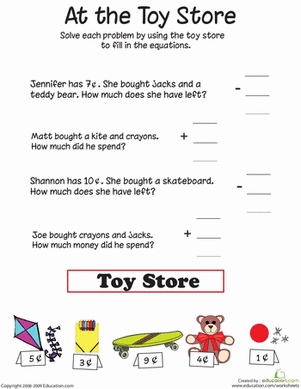At the Toy Store: Addition and Subtraction
Worksheet
At the Toy Store: Addition and Subtraction
Looking for a worksheet to help your child with his maths skills? This printable is all about solving word problems at the toy store.
Year 2
Maths
Worksheet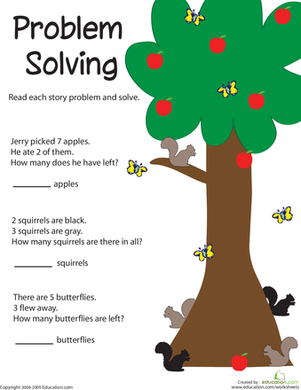Problem Solving: Adding Apples
Worksheet
Problem Solving: Adding Apples
Even in nature, solving story problems is important. How many apples will be left on a tree if seven are picked?
Year 2
Maths
Worksheet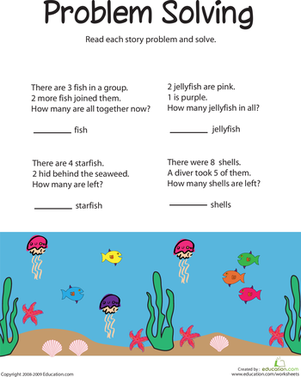Problem Solving: Subtracting Sea Life
Worksheet
Problem Solving: Subtracting Sea Life
Help your child with his maths skills with this printable worksheet that asks him to develop a logical sequence for baking a cake.
Year 2
Maths
Worksheet

## Lesson Plans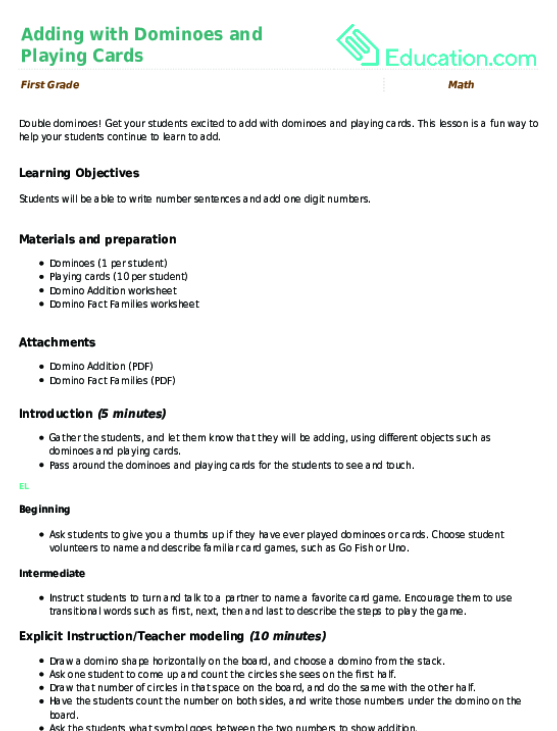Adding with Dominoes and Playing Cards
Lesson plan
Adding with Dominoes and Playing Cards
Double dominoes! Get your students excited to add with dominoes and playing cards. This lesson is a fun way to help your students continue to learn to add.
Year 2
Maths
Lesson planArt Subtraction
Lesson plan
Art Subtraction
Practise subtraction with art! Help early learners grasp the concept of "taking away" using drawings. This lesson helps students work toward subtraction with numerals, and the mastery of simple shapes.
Year 2
Maths
Lesson planAddition: Over in a Flash
Lesson plan
Addition: Over in a Flash
Encourage your students to take part in some friendly competition with this game that has students practise their addition skills with flashcards.
Year 2
Maths
Lesson planLesson plan
Use a sweet treat to help students do hands-on maths! Students will practise their addition skills in this yummy activity.
Year 2
Maths
Lesson plan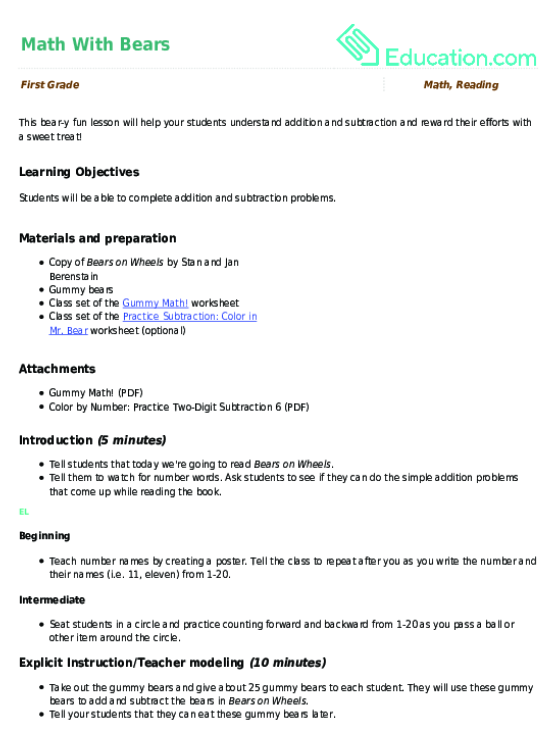Maths With Bears
Lesson plan
Maths With Bears
This bear-y fun lesson will help your students understand addition and subtraction and reward their efforts with a sweet treat!
Year 2
Lesson planActive maths
Lesson plan
Active maths
Have a class that can't sit still long enough to practise their addition? This activity puts maths and PE together to help your kids add up to 20 while being active!
Year 2
Maths
Lesson plan

## Workbooks

No workbooks found for this common core node.

## Games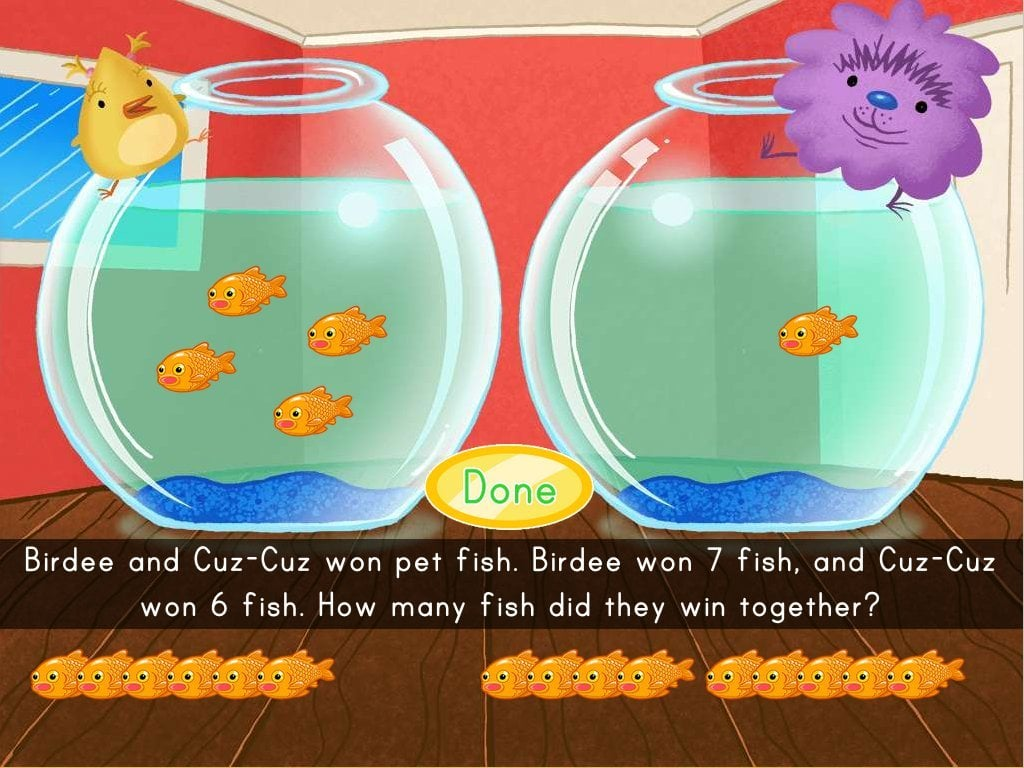Game
Students help Birdee and Cuz-Cuz count up all the fish they won in this addition game.
Year 2
Maths
Game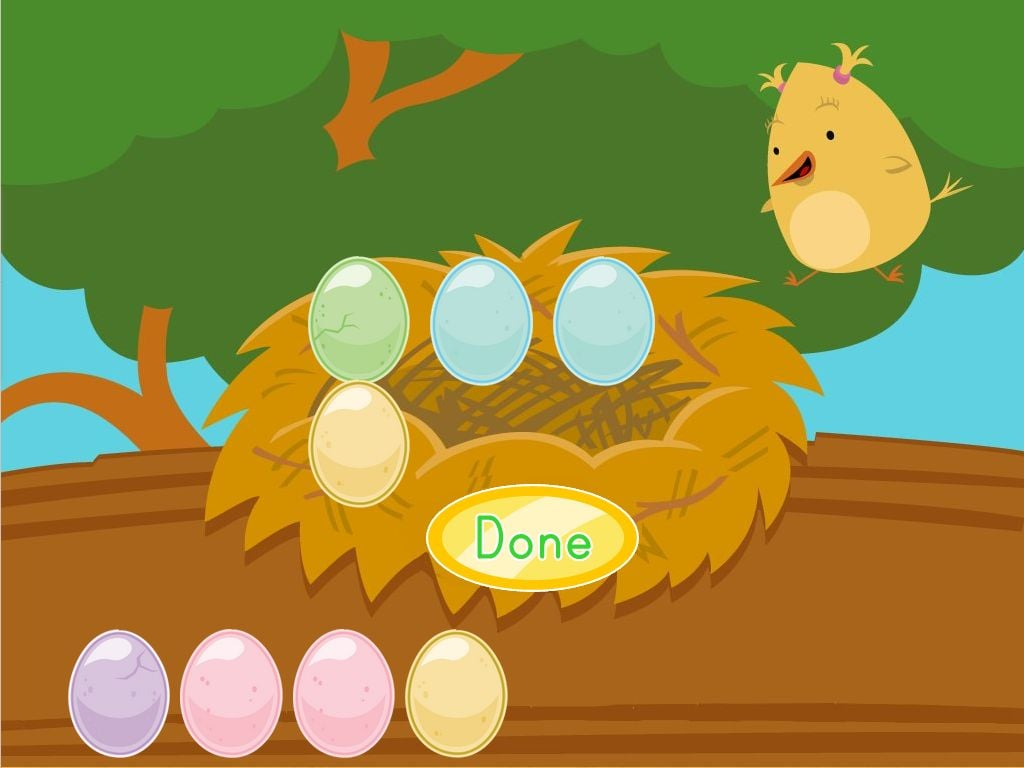Adding Eggs with Birdee
Game
Adding Eggs with Birdee
Your kid will add the right number of eggs to the nest to practise addition facts.
Year one
Maths
Game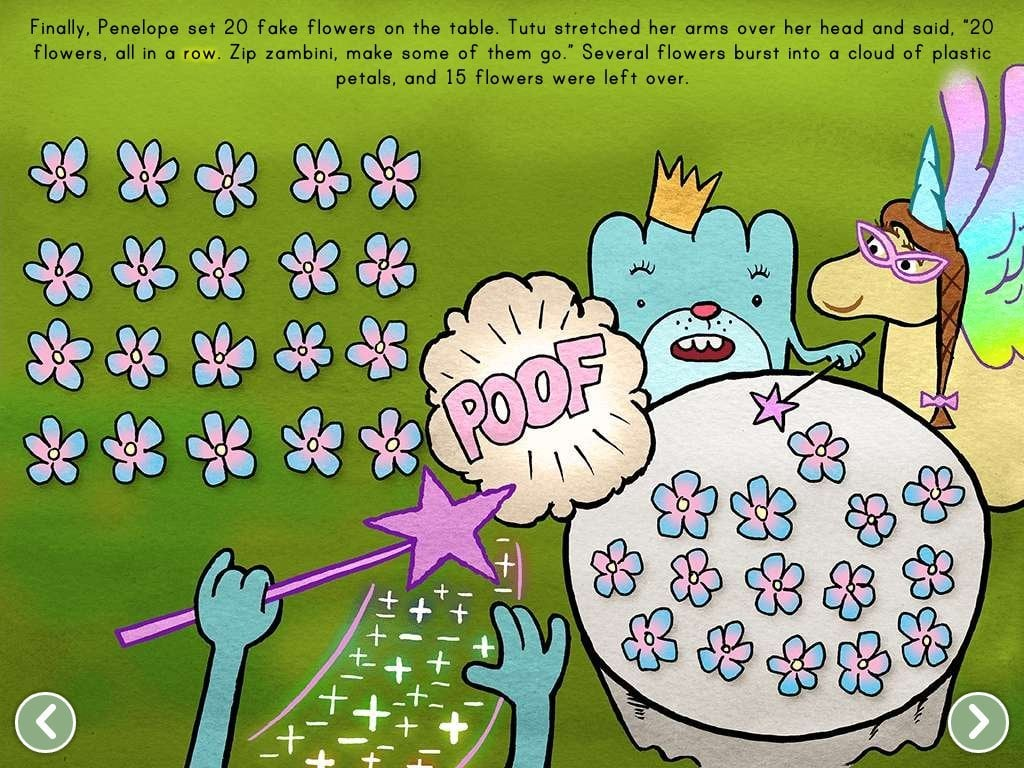Magical Subtraction
Story
Magical Subtraction
Enjoy some maths magic with this fun story that introduces the concept of subtraction.
Year 2
Maths
Story

## Exercises

No exercises found for this common core node.

What could we do to improve Education.com?

Please note: Use the Contact Us link at the bottom of our website for account-specific questions or issues.

What would make you love Education.com?